# Showing items from data structures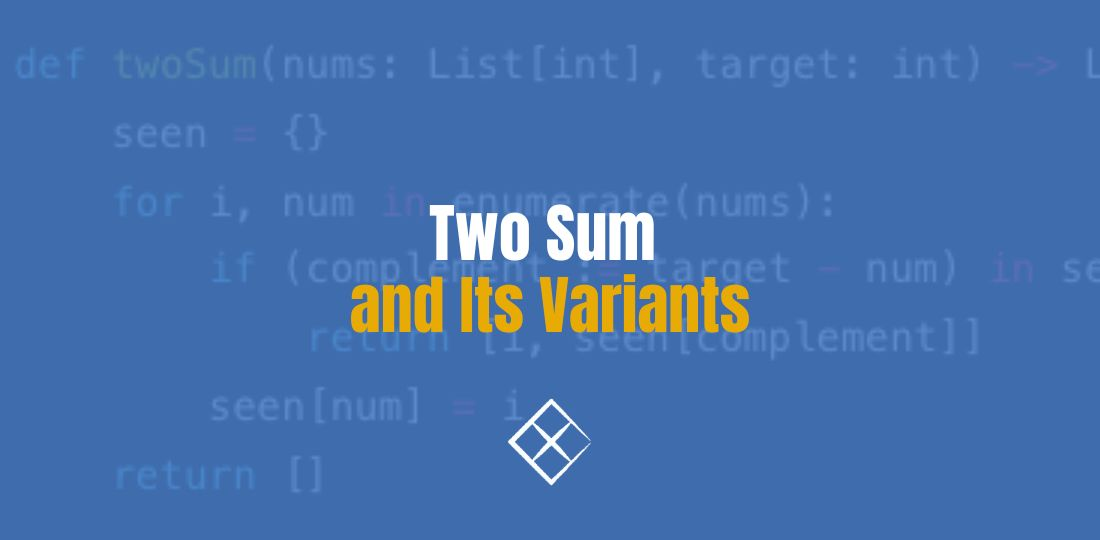### Two Sum and Its Variants

Problem Statement Given an array of integers nums and an integer target, return indices of the two …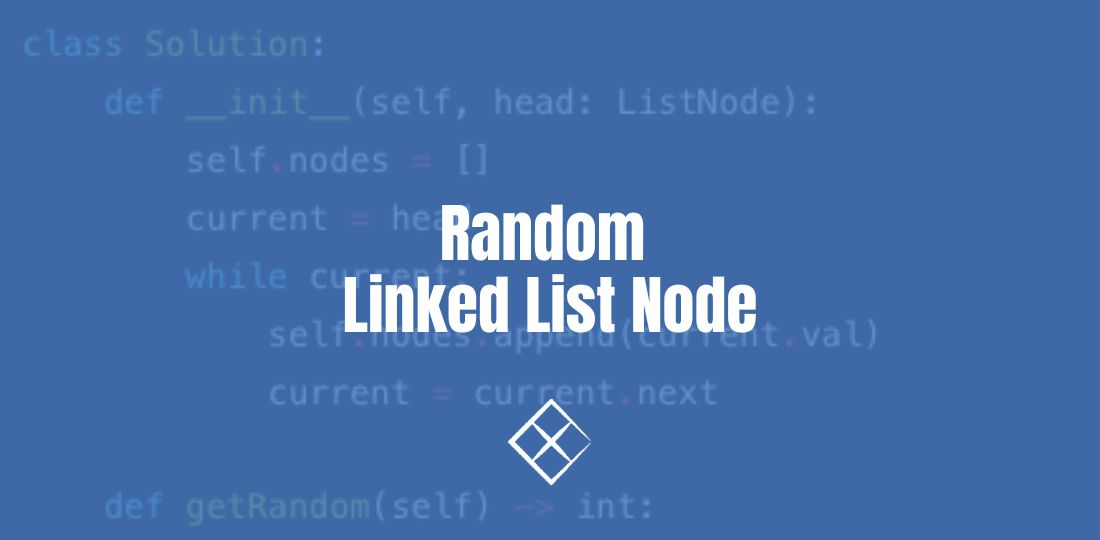Problem Statement Given a singly linked list, return a random node’s value from the linked …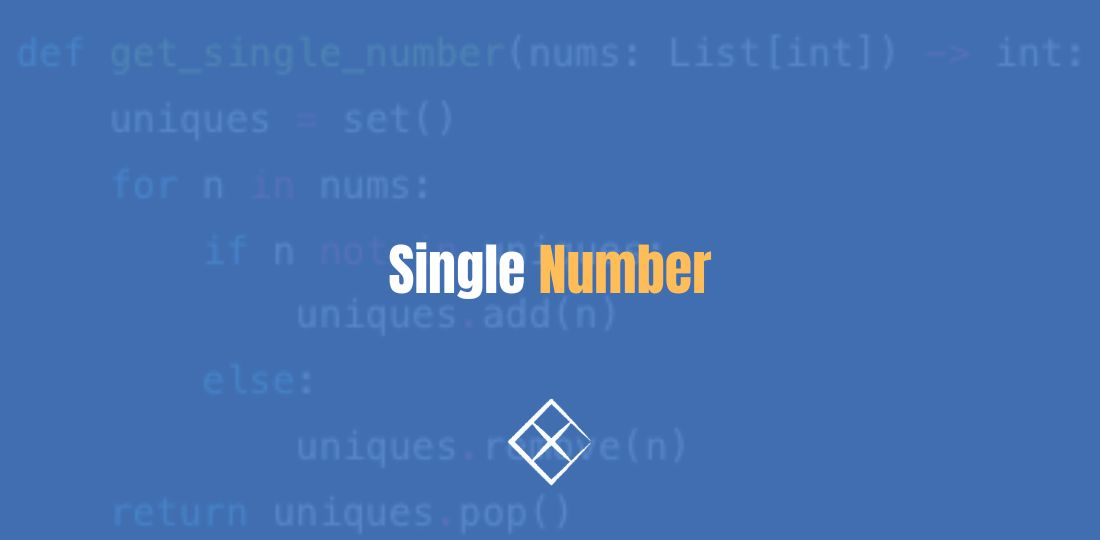### Single Number

Single Number Given a non-empty array of integers nums, every element appears twice except for one. …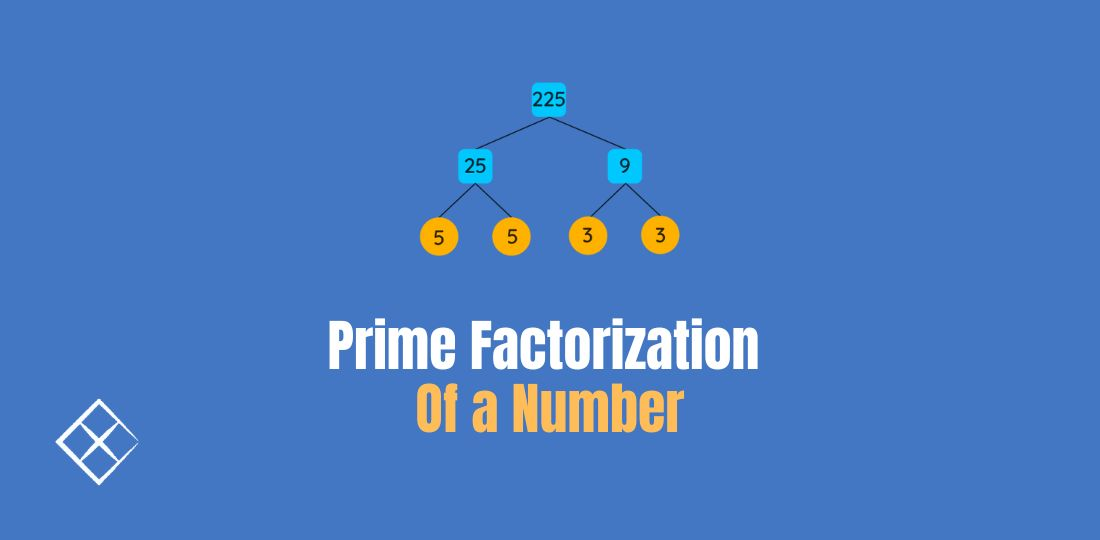### Prime Factorization of a Number

Problem Statement How do we find all the prime factors of a given number? For example, when n = 12, …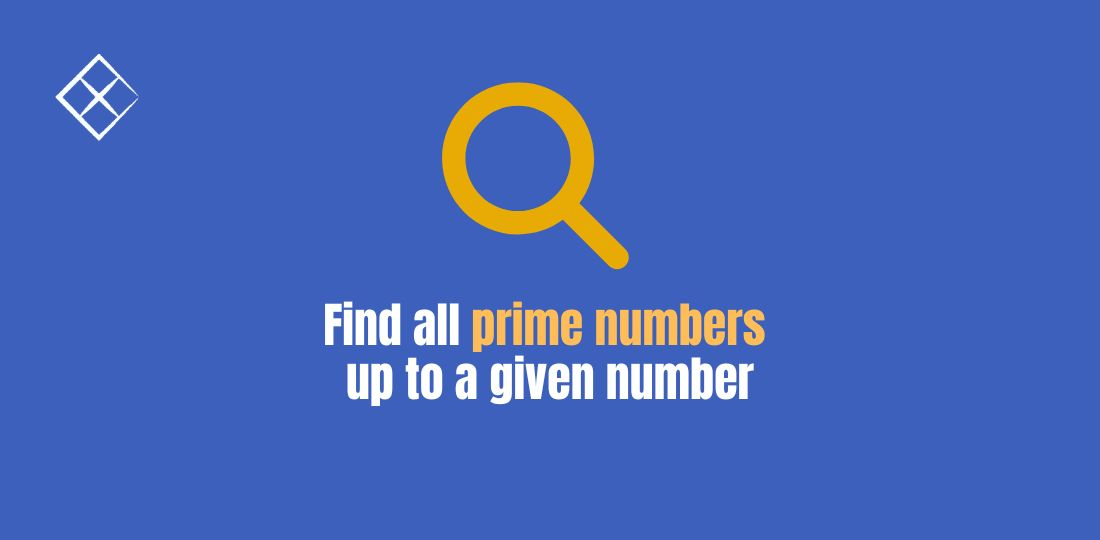### Find all prime numbers up to a given number

Problem Statement How do we find all the prime numbers up to a given number? For example, when n = …